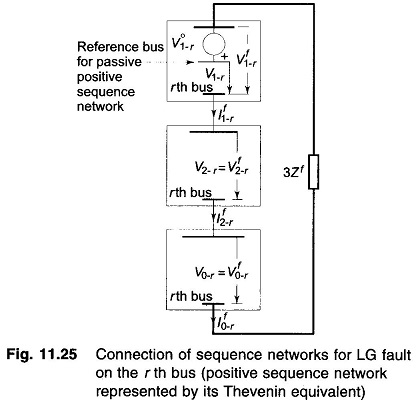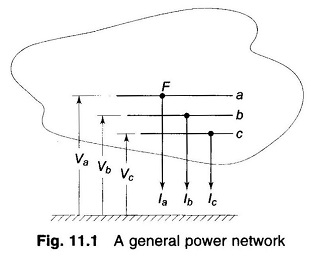# Unsymmetrical Fault Analysis

## Bus Impedance Matrix Method for Analysis of Unsymmetrical Shunt FaultsBus Impedance Matrix Method for Analysis of Unsymmetrical Shunt Faults: Bus Impedance Matrix Method of fault analysis, given for symmetrical faults  can be easily extended to the case of unsymmetrical faults. Consider for example an LG fault on the rth bus of a n-bus system. The connection of sequence networks to simulate the fault is …

## Symmetrical Component Analysis of Unsymmetrical FaultsSymmetrical Component Analysis of Unsymmetrical Faults: Symmetrical Component Analysis of Unsymmetrical Faults – Consider a general power network shown in Fig. 11.1. It is assumed that a shunt type fault occurs at point F in the system, as a result of which currents Ia, Ib, Ic flow out of the system, and Va, Vb, Vc …

## Types of Unsymmetrical faults in Power System

Types of Unsymmetrical faults in Power System: There are two types of unsymmetrical faults in power system namely, Shunt Type Fault: Single Line to Ground Fault (LG) Line to Line Fault (LL) Double Line to Ground Fault (LLG) Series Type Fault: (i) Open conductor (one or two conductors open) fault. It was stated, that a three-phase …

Scroll to Top